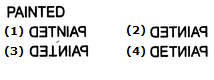# Non Verbal Reasoning - Mirror Images - Discussion

### Discussion :: Mirror Images - Section 1 (Q.No.15)

In each of the following questions you are given a combination of alphabets and/or numbers followed by four alternatives (1), (2), (3) and (4). Choose the alternative which is closely resembles the mirror image of the given combination.

15.

Choose the alternative which is closely resembles the mirror image of the given combination.[A]. 1 [B]. 2 [C]. 3 [D]. 4

Explanation:

No answer description available for this question.

 Pratik said: (Nov 11, 2018) 2 and 4 seems the same. Which is the correct answer?

 Yogananda Naidu said: (Jun 9, 2020) @Pratik. 2 is the correct answer, in 4 E is wrong. Note it.

 Study said: (Sep 15, 2021) B is the correct answer because in 1 N is not changed in 3 T is upside down in 4 E is not changed. So, [B] 2 is the answer.

 Study said: (Sep 15, 2021) B is the correct answer because in 1 N is not changed in 3 T is upside down in 4 E is not changed. So, [B] 2 is the answer.